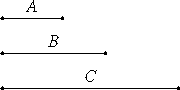# Proposition 29

If an odd number is multiplied by an odd number, then the product is odd.

Let the odd number A multiplied by the odd number B make C.I say that C is odd.

Since A multiplied by B makes C, therefore C is made up of as many numbers equal to B as there are units in A. And each of the numbers A and B is odd, therefore C is made up of odd numbers, the multitude of which is odd. Thus C is odd.

Therefore, if an odd number is multiplied by an odd number, then the product is odd.

Q.E.D.

## Guide

With the completion of this proposition, the study of addition, subtraction, and multiplication of even and odd numbers is also completed. There remain a few more propositions about even and odd numbers.